# sklearn调包侠之逻辑回归### 算法原理

##### 正则化

• L1范数
L1范数作为正则化项，会让模型参数θ稀疏话，就是让模型参数向量里为0的元素尽量多。L1就是在成本函数后加入: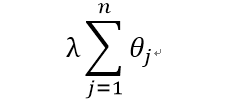• L2范数
而L2范数作为正则化项，则是让模型参数尽量小，但不会为0，即尽量让每个特征对预测值都有一些小的贡献。L2就是在成本函数后加入: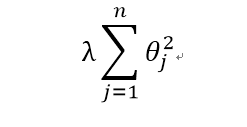### 实战——乳腺癌检测

##### 数据导入

from sklearn.datasets import load_breast_cancer
print(cancer.DESCR)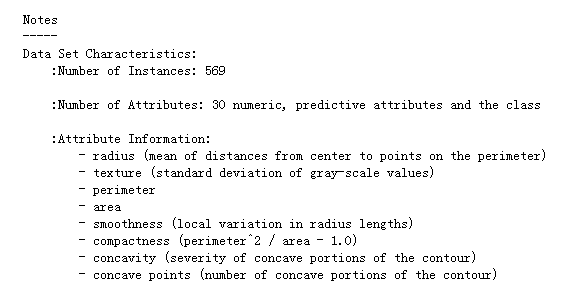##### 切分数据集
X = cancer.data
y = cancer.target

from sklearn.model_selection import train_test_split
X_train, X_test, y_train, y_test = train_test_split(X, y, test_size=0.2, random_state=33)

##### 模型训练与评估

• penalty：设置正则化项，其取值为'l1'或'l2'，默认为'l2'。
• C：正则化强度，C越大，权重越小。
from sklearn.linear_model import LogisticRegression

model = LogisticRegression()
model.fit(X_train, y_train)
model.score(X_test, y_test)

# result
# 0.94736842105263153


model2 = LogisticRegression(penalty='l1')
model2.fit(X_train, y_train)
model2.score(X_test, y_test)

# result
# 0.95614035087719296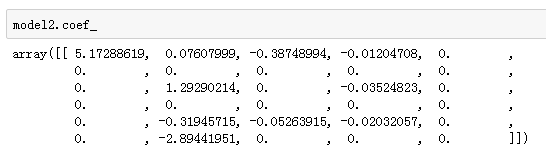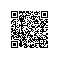使用钉钉扫一扫加入圈子
+ 订阅